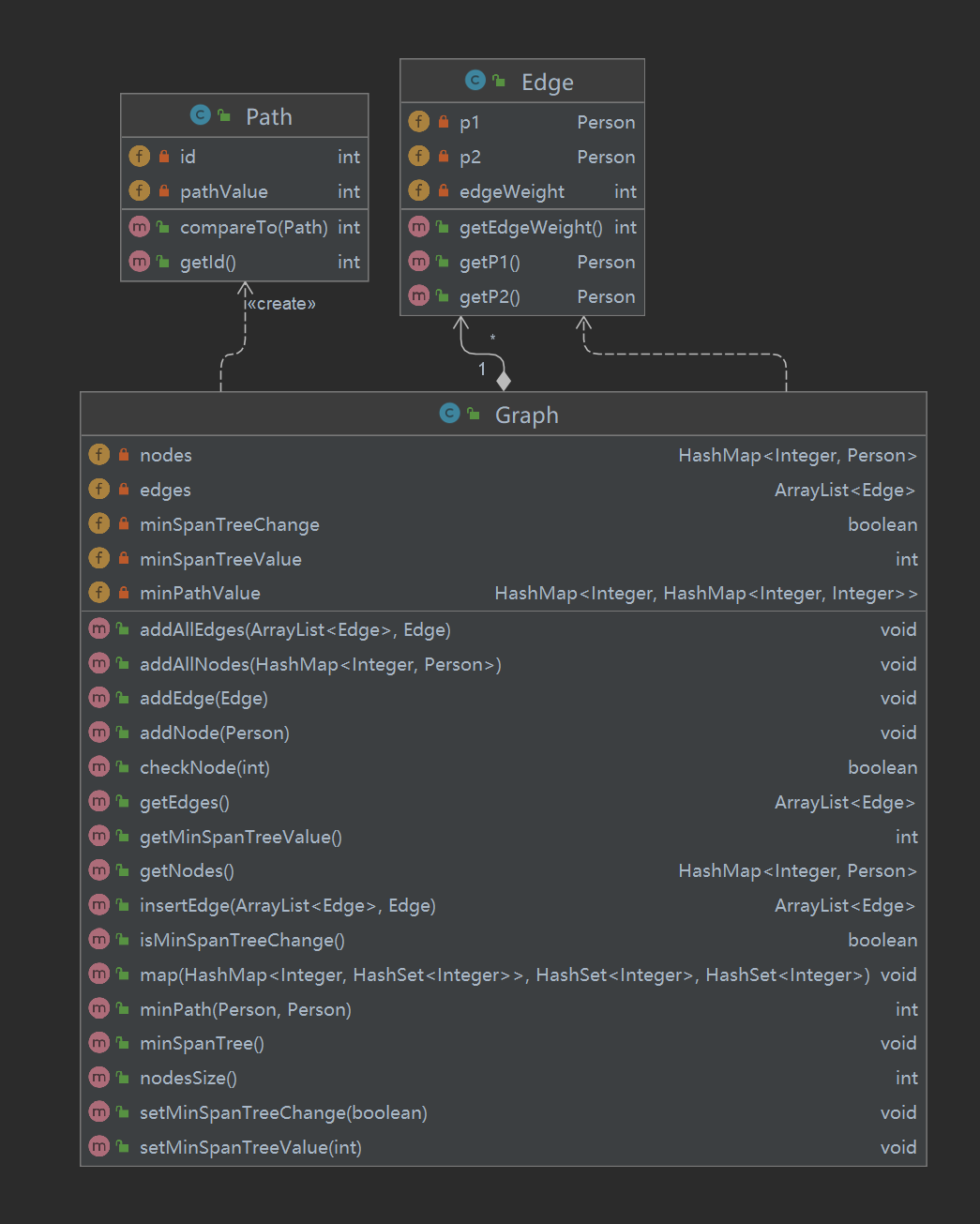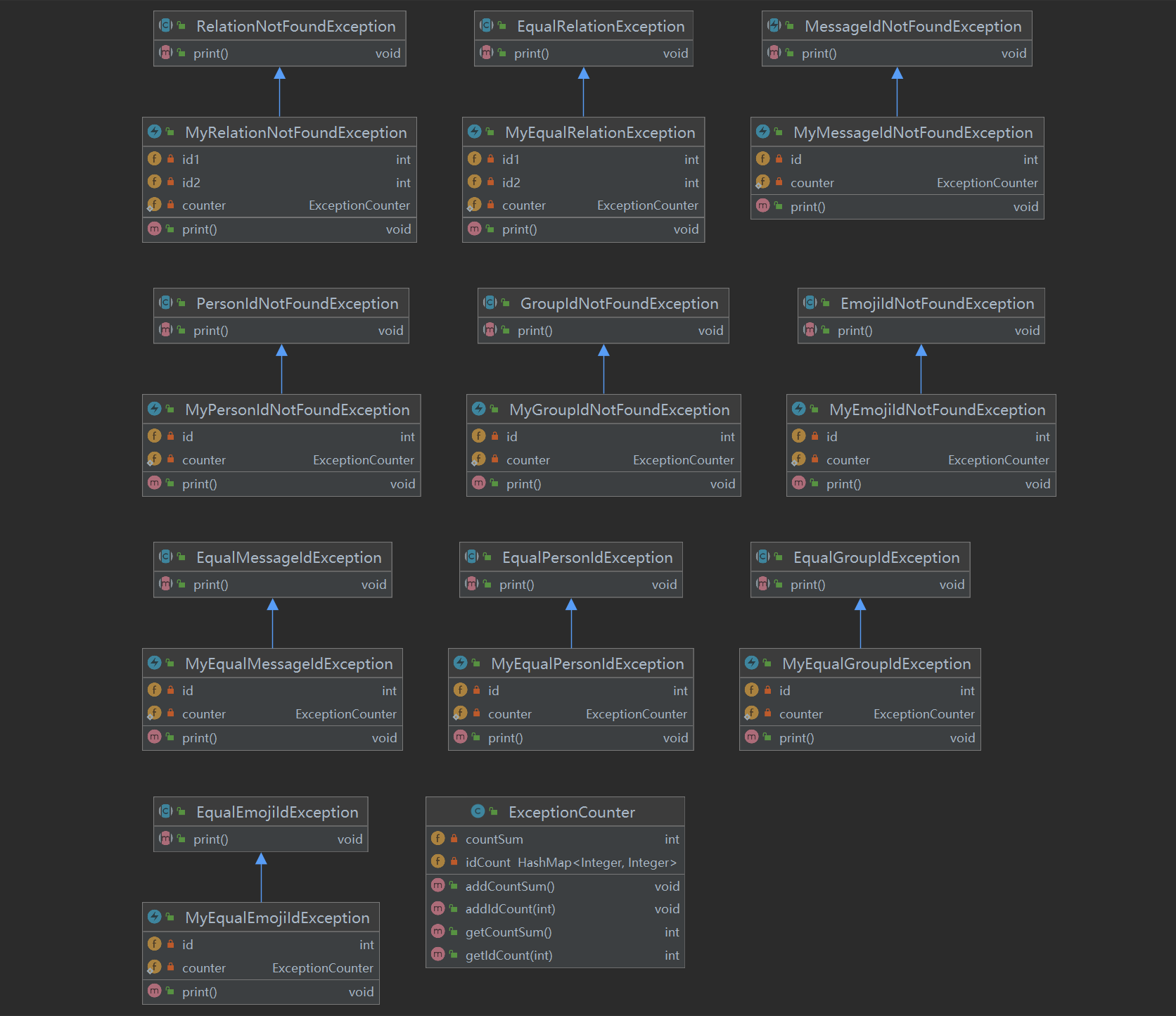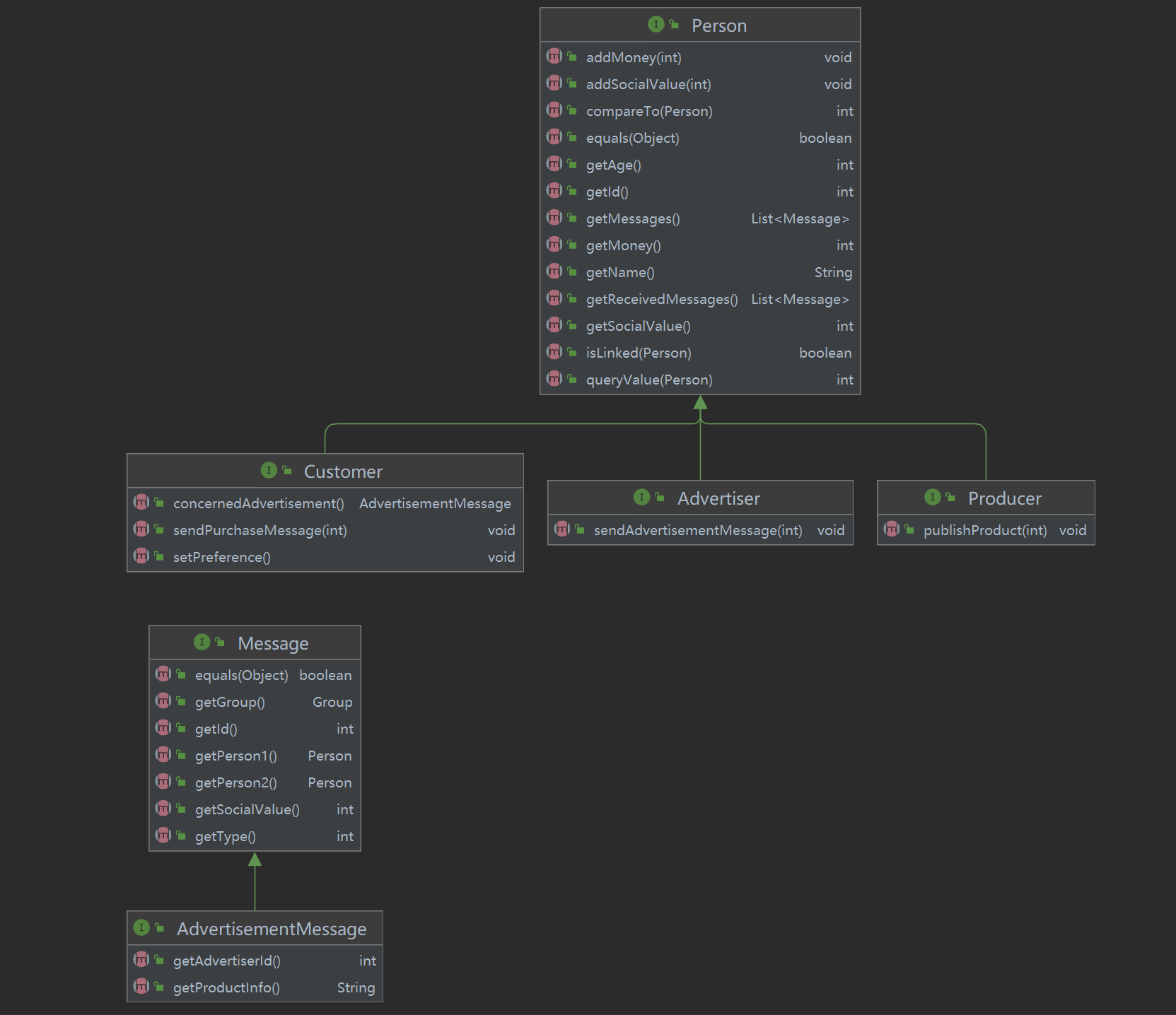## BUAA-OO-第三单元总结

### 二、方案实现

#### 1、第一次作业

public void addToRelationGroup(Person p1, Person p2) {
int indexP1 = -1;
int indexP2 = -1;
int flag = 0;
for (int i = 0; i < relationGroup.size(); i++) {
if (relationGroup.get(i).containsKey(p1.getId())) {
indexP1 = i;
flag++;
}
if (relationGroup.get(i).containsKey(p2.getId())) {
indexP2 = i;
flag++;
}
if (flag == 2) {
break;
}
}
if (indexP1 == -1 && indexP2 == -1) {
HashMap<Integer, Person> group = new HashMap<>();
group.put(p1.getId(), p1);
group.put(p2.getId(), p2);
} else if (indexP1 != -1 && indexP2 == -1) {
relationGroup.get(indexP1).put(p2.getId(), p2);
} else if (indexP1 == -1) {
relationGroup.get(indexP2).put(p1.getId(), p1);
} else {
if (indexP1 != indexP2) {
HashMap<Integer, Person> group1 = relationGroup.get(indexP1);
HashMap<Integer, Person> group2 = relationGroup.get(indexP2);
relationGroup.remove(group1);
relationGroup.remove(group2);
if (group1.size() <= group2.size()) {
group2.putAll(group1);
} else {
group1.putAll(group2);
}
}
}
}


#### 2、第二次作业Edge类记录两边结点Person以及边的权值，Graph类记录了一个连通图中的所有结点，并且采用kruskal算法来生成最小生成树，算法实现为把边按照权值大小排序，然后从最小的边开始选择，把两边结点加入到最小生成树的结点集合中，当加入的边其两边结点不都在最小生成树的结点集合中时，该边可加入到最小生成树，这样在构造过程中就保证了不会成环。即每次选择最小边，并且保证已经选择的边不会成环。为了减少算法的复杂度，在维护连通图中存储的边时，采取了这样的策略，经过一次最小生成树计算之后，边集中只保留最小生成树的边，合并两个图时，若两个图都是未更新的并且已经计算过最小生成树，那么合并这两个图只需要把两个图的边集合并再加上新增的边即可。

qgvs指令通过分析可得，如果按照JML的描述，无论采取ArrayList（O（mn^2））存储还是HashMap（计算hash值耗费时间）存储都有较高的复杂度。但如果我们遍历每个人的acquaintance集合，那么当每个人的acquaintance集合较小时，复杂度会下降很多，而测试点中，为稠密图的可能性并不大，并且必须当group中的人数小于acquaintance中的人数，这个策略才会耗时更多。

#### 3、第三次作业#### 4、架构设计，图模型构建维护总结### 五、Network扩展/*@ public normal_behavior
@ requires containsMessage(id)
@ assignable messages;
@ assignable people[*].messages
@ ensures !containsMessage(id) && messages.length == \old(messages.length) - 1 &&
@ (\forall int i;0 <= i && i < \old(messages.length) && \old(messages[i].getId()) != id;
@ (\exists int j;0 <= j && j < messages.length;messages[j].equals(\old(messages[i]))));
@ ensures (\forall int i;0 <= i && i < people.length && this.isLinked(people[i]);
@ (\forall int j;0 <= j && j < \old(people[i].getMessages().size());
@ people[i].getMessages().get(j + 1) == \old(people[i].getMessages().get(j))) &&
@ people[i].getMessages().get(0).equals(\old(getMessage(id))) &&
@ people[i].getMessages().size() == \old(people[i].getMessages().size()) + 1);
@ ensures (\forall int i;0 <= i && i < people.length && !this.isLinked(people[i]);
@ people[i].getMessages() == \old(people[i].getMessages()));
@ also
@ public exceptional_behavior
@ signals (MessageIdNotFoundException e) !containsMessage(id);
*/

/*@ public normal_behavior
@ requires !containsProduct(id)
@ assignable products;
@ ensures (\forall int i;0 <= i && i <= \old(products.length);
@ (\exists int j;0 <= j && j <= products.length;products[j].equals(\old(products[i]))));
@ ensures (\exists int i;0 <= i && i < products.length;products[i].equals(getProduct(id)));
@ ensures products.length == \old(products.length) + 1;
@ also
@ public exceptional_behavior
@ signals (EqualProductIdException e) containsProduct(id);
*/
void publishProduct(int id);

/*@ public normal_behavior
@ assignable messages;
@ ensures (\forall int i;0 <= i && i <= \old(messages.size()) && message[i].getId != id;
@ (\exists int j;0 <= j && j <= messages.size();messages[j] == \old(messages[i])));
@ ensures (\forall int i;0 <= i && i <= messages.size();message[i].getId != id);
@ ensures messages.size() = \old(message.size()) - 1;
@ ensures (\forall int i;0 <= i && i <= \old(getPerson(advertiserId).getMessages().size());